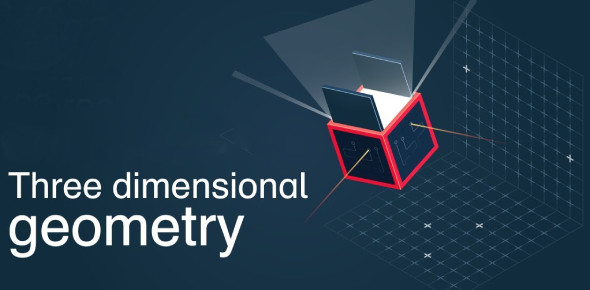Three Dimensional Geometry Test! Trivia Quiz

15 Questions | Total Attempts: 283Settings.

• 1.
Find the length of the perpendicular drawn from the origin to the plane 2x-3y + 6z + 21 = 0.
• A.

3

• B.

4

• C.

5

• D.

6

• 2.
Write the direction cosines of the vector -2i + j – 5k
• A.
• B.
• C.
• D.
• 3.
Find the coordinates of the point, where the line  intersects the plane x – y + z – 5 = 0. Also find the angle between the line & the plane.
• A.
• B.
• C.
• D.
• 4.
If a line makes angles 900, 1350, 450 with x, y, and z-axes respectively, find its direction cosines.
• A.
• B.
• C.
• D.
• 5.
Find the vector equation of the plane which contains the line of intersection of the planes & which is perpendicular to the plane
• A.
• B.
• C.
• D.
• 6.
There are two lines in which one line is passing through the points (4, 7, 8) (2, 3, 4) & another line is passing through the points (-1, -2, 1), (1, 2, 5). What is true regarding these lines?
• A.

AB is parallel to CD

• B.

AB is perpendicular to CD

• C.

AB is inclined to CD at certain angle

• D.

None of these

• 7.
Find the angle between the following pair of lines:
• A.
• B.
• C.
• D.
• 8.
Find the Cartesian equation of the line which passes through the point (-2, 4, -5) and is parallel to the line:
• A.
• B.
• C.
• D.
• 9.
Find the equation of the plane through the line of intersection of the planes x + y + z = 1 and 2x + 3y + 4z = 5 which is perpendicular to the plane x – y + z = 0
• A.

X - z - 2 = 0

• B.

X -y + 2 = 0

• C.

X - z + 2 = 0

• D.

Y - z + 2 = 0

• 10.
Find the coordinates of the point where the line through (3, -4, -5) and (2, -3, 1) crosses the plane, passing through the points (2, 2, 1), (3, 0, 1) and (4, -1, 0).
• A.

(1, -2, 7)

• B.

(1, 2, 7)

• C.

(1, -2, 5)

• D.

(2, -2, 7)

• 11.
Find the Cartesian equation of the plane which contains the line of intersection of the planes
• A.

33x +45y + 50z - 41 = 0

• B.

33x + 45y + 50z - 41 = 0

• C.

X + 45y + 50z - 41 = 0

• D.

33x + y + 50z - 41 = 0

• 12.
Show that the lines are intersecting. Hence find their point of intersection.
• A.

(-1, -6, 12)

• B.

(-1, 6, -12)

• C.

(1, -6, -12)

• D.

(-1, -6, -12)

• 13.
Find the vector equation of the plane through the points (2, 1,-1) and (-1, 3, 4) and perpendicular to the plane x -2y+ 4z = 10.
• A.
• B.
• C.
• D.
• 14.
Find the shortest distance between the lines:
• A.
• B.
• C.
• D.
• 15.
Find the equation of the plane passing through the line of intersection of the planes whose perpendicular distance from origin is unity.
• A.
• B.
• C.
• D.
Related TopicsBack to top